All submissions of the EM system will be redirected to Online Manuscript Submission System. Authors are requested to submit articles directly to Online Manuscript Submission System of respective journal.

# Research

, Volume: 11( 3) DOI: 10.37532/2320-6756.2023.11(3).329

## The Mirror Reversal Mechanism on Black Hole Collapse and Singularity Eruption in Cosmic Continuum

*Correspondence:
Xijia Wang
Geophysical Society of Hunan Province, Changsha, 410000, China,
E-mail:
xijiawang@hunnu.edu.cn

Received date: 3-March-2023, Manuscript No. tspa-23-90727; Editor assigned: 5-March-2023, Pre-QC No. tspa-23-90727 (PQ); Reviewed: 18- March-2023, QC No. tspa-23-90727 (Q); Revised: 20-March-2023, Manuscript No. tspa-23-90727 (R); Published: 23-March-2023, DOI. 10.37532/2320-6756.2023.11(3).329

Citation: Wang X. The Mirror Reversal Mechanism on Black Hole Collapse and Singularity Eruption in Cosmic Continuum. J. Phys. Astron.2023;11(3):329.

### Abstract

In the Big Bang Theory and the Black Hole Theory, the existing laws of physics all fail at the singularity, and the singularity has become a blind spot in the existing scientific theories. In the axioms system of cosmic continuum, the universe was not created by an instantaneous explosion, but by the continuous eruption of singularities. As long as the singularity is still erupting, the total amount of the universe will continue to increase. The so-called dark energy is actually the gravitational field energy erupted by the singularity. The expansion force of dark energy is generated by the outward eruption of singularity. The cosmic system collapse into a Schwarzschild black hole under the action of a strong gravitational field, and the Planck spheres at the center of the black hole continues to collapse into dark mass bodies, forming dark celestial body and singularity. The Schwarzschild radius is the upper limit of a black hole, and the Planck sphere is the lower limit of a black hole. The singularity is the conversion point between the old and new cosmic systems. The singularity erupts the Planck spheres under the action of a strong gravitational field, and the Planck spheres expands outward to form a new cosmic system. The Planck sphere is both the end of the old cosmic system and the starting point of the new cosmic system. The black hole collapse and the singularity eruption are mirror images of each other

### Keywords

Cosmic continuum; Singularity theory; Planck sphere; Dark mass; Dark energy

### Introduction

The Big Bang theory believes that the observable universe was created by a big bang that occurred at a dense and hot singularity about 13.8 billion years ago. With the continuous expansion after the Big Bang, the universe evolves in temperature from hot to cold and matter from dense to sparse .

In modern cosmology, black holes are formed by the collapse of celestial bodies or cosmic systems.

The Schwarzschild radiusis the upper limit of the black hole. Where G is the gravitational constant, c is the speed of light, and M is the mass of the celestial body. At the center of the black hole, it will collapse to form a singularity of infinite mass, density, temperature, and space-time curvature, and infinitely small volume.

However, both the Big Bang theory and the black hole theory face the same paradox: a singularity is a point that exists and does not exist, and all known laws of physics fail at the singularity.

The Planck unit theory holds that the Planck mass mp , the Planck energy Ep , the Planck time tp , and the Planck length lp , have specific cosmological significance [2-10]. In the Planck unit system, Planck mass, Planck energy, Planck time, Planck length. Where h is Planck constant.

Cosmic continuum is a mathematical continuum theoretical model of the universe [11-22]. Deduced from the axiom system of the cosmic continuum: the universe was not created by an instantaneous explosion, but by the continuous eruption of singularities. As long as the singularity is still erupting, the universe will not stop expanding.

### Derivation

Cosmic Continuum Theory believes that the universe is a continuum composed of mass body, energy body, dark mass body and space, time, dark space. Space, time, and dark space are the existence dimensions of mass bodies, energy bodies, and dark mass bodies, respectively. The mass body, the energy body, and the dark mass body are respectively composed of particles, quantum and dark particles.

In Cosmic continuum, in addition to known celestial bodies such as planets, stars, and black holes, there is also a dark celestial body in the universe. Its existence quantity is dark mass and its existence dimension is dark space.

Concept 1: Planck sphere refers to a space spheres whose diameter is the Planck length lp .

Concept 2: Black hole refers to a celestial body with the Schwarzschild radius Rs , as the upper limit and the Planck sphere as the lower limit.

Concept 3: Dark celestial bodies refer to celestial bodies composed of dark mass bodies. The following lemmas are all quoted from .

Lemma 1: When the mass of particles reaches Planck mass mp , the particles are converted into quantum and the mass is converted into energy (proof see ).

Lemma 2: When quantum energy reaches Planck energy Ep , quantum is transformed into dark particles and energy is converted into dark mass (proof see ).

Lemma 3: Planck mass mp is equivalent to Planck energy : Ep : mp ≡ Ep (proof see ).

Lemma 4: Planck time tp is equivalent to Planck length : lp : tp ≡ lp (proof see ).

Corollary 1: When the amount of Planck sphere reaches e mpEp , the Planck sphere collapses into dark particles. Proof: If the existence quantity of Planck sphere reaches emp , according to Lemma 1, when the mass of the particle reaches Planck mass, that is, emp , the particle is transformed into quantum, and mass mp will be transformed into energy. According to Lemma 3, the Planck mass mp is equivalent to the Planck energy Ep : mpEp . According to Lemma 2, when the energy of a quantum reaches Planck energy Ep , the quantum is transformed into dark particles, and the energy is transformed into dark mass. If the existence quantity of Planck sphere reaches eEp , according to Lemma 2, when the energy of the quantum reaches the Planck energy Ep , the quantum is transformed into dark particles, and the energy is transformed into dark mass. In summary, when the existence quantity of Planck sphere reaches empEp , the Planck sphere collapse into dark particles. Q.E.D.

Corollary 1 shows that the Planck sphere is the lower limit of a black hole, and the Planck mass and Planck energy are the limit mass and limit energy of the Planck sphere, respectively.

Concept 4: Planck particles are Planck spheres that collapse into dark particles.

According to Corollary 1, the existence of Planck particles is the dark mass D , DmpEp.

Concept 5: Planck dark mass Dp refers to the dark mass of Planck particles.

According to Concept 4, Planck dark quality Dp = DmpEp .

Concept 6: Planck existence quantity ep refers to the existence quantity of Planck particles.

According to Corollary 1 and Concept 4, Concept 5, Planck existence quantity epmpEpDp .

Corresponding to the Planck unit of the existing quantity, the Planck dimension metric Wp can also be defined as the dimension metric of the Planck particle, the Planck dark space metric gp is the dark space degree of the Planck particle, and Wptplpgp .

Corollary 2: The limit density of Planck sphere isProof: According to Corollary 1, the limit mass-energy of the Planck sphere is emaxmpEp .

According to Concept 6, epemax . According to Concept 1, the Planck sphere refers to a sphere with a diameter of Planck lengthso the volume of the Planck sphere is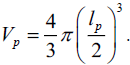Let e be the existence quantity of Planck spheres, then the limit density of Planck spheresCorollary 3: In the process of a black hole collapse, every Planck time tp collapses a Planck sphere, the existence quantity collapse speed is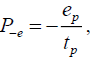and the negative sign " - " indicates the collapse.

Proof: According to Concept 1, Planck sphere refers to a space spheres whose diameter is the Planck length lp . According to Lemma 4, Planck time tp is equivalent to Planck length lp : tplp . Therefore, the collapse of a Planck sphere is equivalent to the collapse of a Planck time. According to Corollary 1, when the Planck sphere reaches limit existence quantity emaxmpEp , the Planck sphere collapses into a dark particle. According to Concept 4, Planck particle is a Planck sphere that collapses into a dark particle, and its existence quantity is Demax . According to Concept 5, DpDemax . According to Concept 6, Planck existence quantity epDp . Once the Planck sphere at the center of the black hole begins to collapse toward the dark mass, it will continue to collapse until it no longer collapses. Therefore, during the collapse of the black hole, every Planck time tp collapses a Planck sphere, and the velocity of the existence quantity collapse isthe negative sign " - " means collapse. Q.E.D.

Corollary 4: In the process of a black hole collapse, the space collapses in a spherical shape with the Planck sphere as the center, and the collapse radius is R = -nlp , and the negative sign " - " means collapse.

Proof: Because the Planck sphere is spherical, when the Planck sphere collapses, the space also collapses in a spherical shape with the Planck sphere as the center. That is, in a Planck time tp , the collapsed space around the sphere is the Planck length lp , so the space collapse radius is r = lp . According to Corollary 3, during the collapse of the black hole, every Planck time tp collapses a Planck sphere, so the space collapse radius is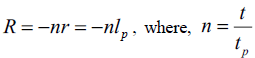represents the nth Planck time, t represents the collapse time, and the negative sign " - " means collapse. Q.E.D.

Concept 7: Singularity refers to a dark celestial body formed by the collapse of a black hole.

Concept 7 shows that the singularity is a special kind of dark celestial body.

Corollary 5: In the process of a singularity eruption, every Planck time tp erupts a Planck sphere, and the eruption velocity of the existence quantity is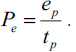Proof: Same as Corollary 3, just replace the black hole collapse process with the singularity eruption process. Q.E.D.

Corollary 6: In the process of a singularity eruption, the space expands in a spherical shape with the Planck sphere as the center, and the expansion radius is R = nlp , whererepresents the nth and t represents the expansion time.

Proof: Same as Corollary 4, just replace collapse with expansion. Q.E.D.

### Result

Mirror reversal theorem

The black hole collapse and the singularity eruption are mirror reversals of each other.

Proof: According to Corollary 3 and Corollary 4: In the process of a black hole collapse, every Planck time tp collapses a Planck sphere, the existence quantity collapse speed is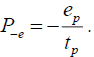The space collapses in a spherical shape with the Planck sphere as the centre, and the collapse radius is R = -nlp , and the negative sign " - " means collapse. According to Corollary 5 and Corollary 6: In the process of a singularity eruption, every Planck time tp erupts a Planck sphere, and the eruption velocity of the existence quantity is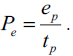The space expands in a spherical shape with the Planck sphere as the center, and the expansion radius is R = nlp, wherethe nth and t represents the expansion time. The black hole collapse and the singularity eruption are like the same process inside and outside the mirror, so the black hole collapse and the singularity eruption are mirror images of each other. Q.E.D.

Mirror reversal theorem shows that the mechanism of the collapse and the expansion of the universe system is: the old universe system collapses into a black hole, and the Planck sphere at the center of the black hole continues to collapse to form a singularity; the singularity erupts the Planck sphere, and the Planck sphere expands to generate a new cosmic system. The Planck sphere is both the end of the old cosmic system and the starting point of the new cosmic system.

It should be pointed out that the mirror inversion theorem is only a mechanism for the collapse and expansion of the universe system, and any universe system is only a component of the entire universe.

### Discussion

We use the mirror reversal theorem to discuss the observable universe.

Let ti = ntp , where n =2i , tp is Planck time, and i is a natural number. Not hard to get, when i = 202 :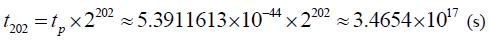Which can be converted to t202 ≈10.98billion years.

According to existing cosmological theories, the age of the observable universe is about 13.8 billion years. t202 is closest to this number, since t203 = t202 ×2 ≈1.96 billion years.

Let's look at the size of the universe formed when the singularity erupted continuously for t202 ≈10.98billion years.

According to corollary 5, when the singularity erupts continuously for t202 ≈109.8billion years, the total existence quantity of the universe formed is: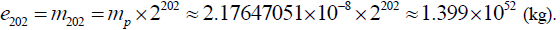According to corollary 6, when the singularity erupts continuously for t202 ≈109.8billion years, the radius of the universe formed is:which can be converted light years.

In December 2011, more than 80 high school students and their teachers in the United States multiplied the Planck length by 2 again and again to 202 times and obtained this result .

Perhaps, there are still many doubts: Where does the increasing mass of the observable universe come from? Why is there a Planck sphere every Planck time? Is the singularity dense and hot or cold and dark? Was the observable universe created by the Big Bang or was it quietly expanding? How do the four interactions occur? Where are the dark matter and dark energy in the universe? [24-42].

Below, let us use the universe continuum and its mirror reversal theory to answer these puzzles one by one.

First, the ever-increasing existence quantity of the observable universe comes from the eruption of singularities. The singularity is formed by the collapse of the old cosmic system. After the old cosmic system collapsed into a black hole, the Planck sphere at the center of the black hole continued to collapse to form a singularity. The existence quantity of singularity is determined by the existence quantity of singularity that the old cosmic system collapses to.

Second, time and space belong to the category of existential dimensions. Since the Planck time is equivalent to the Planck length, and the Planck sphere is a space sphere with the Planck length as the diameter, the black hole collapse and singularity eruption are both a Planck sphere per Planck time , And the existence quantity collapse and eruption speeds, and the space collapse and expansion speeds are uniform.

Third, the singularity is a kind of dark celestial body, and its existential dimension is dark space. Therefore, the singularity does not occupy space, let alone the concepts of density and temperature. The reason why a singularity is considered to be a point that both exists and does not exist, and all known laws of physics fail at the singularity because the singularity is beyond the cognitive scope of the existing theories of physics foundation.

Fourth, the density of the Planck sphere erupted by the singularity is the limit density, which is the maximum density in the observable universe. The temperature of the Planck sphere erupted by the singularity should also be the limit temperature, that is, the Planck temperature. Therefore, the observable universe is a spherical expansion of a dense and hot Planck sphere.

Fifth, after the collapse of the old cosmic system into a singularity, the singularity only has gravitational interaction with the outside world. After the singularity erupts the Planck sphere, during the expansion process, due to the background radiation, quantum fluctuations and other factors in the entire universe, the new cosmic system generates Symmetry Breaking to form positive and negative charges and particles of matter, resulting in electromagnetic interaction, strong and weak interactions and evolve into large-scale cosmic structures.

Sixth, the universe is full of existences in the form of dark mass. Celestial bodies composed of dark masses are dark celestial bodies. Dark objects generally exist in the center of the black hole system. The singularity of the observable universe is also a dark celestial body. Dark energy is the gravitational field energy that fills the universe, and it is the source of all energy in the universe. This is because the singularity continuously ejects gravitational field energy. The gravitational field energy diverges outward with the singularity as the center, thus generating expansion force.

### Conclusion

The discussion in this article shows that the singularity is the conversion point between the old and new cosmic systems. The collapse of the black hole and the eruption of the singularity are mirror images of each other. The Planck sphere is the front of the mirror, and the singularity is the back of the mirror. Celestial bodies or cosmic systems collapse to form black holes, and the Planck sphere at the center of the black hole continuously collapses into dark masses, forming dark celestial bodies and singularities. The singularity erupts the Planck sphere, and the Planck sphere expands with the singularity as the center to form a new cosmic system.

The singularity is not a point with infinite mass, infinite density, infinite temperature, infinite curvature of space-time, and infinitely small volume. A singularity is a kind of dark celestial body, its existence quantity is dark mass, and its existence dimension is dark space. Since the singularity does not occupy space, there is no concept of density, temperature, and curvature of space-time. The dark mass of a singularity is the sum of the existence quantity of black hole collapsed.

The universe is a continuum composed of an infinite number of cosmic systems including the observable universe. The mass and energy of the observable universe are not produced from the void, but transformed from the dark mass of the singularity; time and space are not born out of nothing, but are transformed from the dark space of the singularity. When all the dark mass of the singularity is erupted, the universe will stop expanding under the action of gravity and start collapsing until it returns to the singularity.

The dark energy in the universe is actually the gravitational field energy erupted by the singularity. Since the singularity is composed of dark mass, its contact with the outside world can only be through the gravitational field, so the initial form of singularity eruption is gravitational field energy. The repulsive force of dark energy is formed by the outward divergence of the gravitational field energy erupted by the singularity.

### Data availability statement

The data that support the findings of this study are available from the corresponding author upon reasonable request.

The authors have no relevant financial or non-financial interests to disclose.

### References

Welcome Message

Select your language of interest to view the total content in your interested language

###### Citations : 272

Journal of Physics & Astronomy received 272 citations as per Google Scholar report

#### Indexed In

• Open J Gate
• China National Knowledge Infrastructure (CNKI)
• Cosmos IF
• Directory of Research Journal Indexing (DRJI)
• MIAR
• Secret Search Engine Labs
• Euro Pub
• clarivate-web-of-science-logo-vector
• ICMJE

View More

### 9th International Conference on Physics

Edinburgh, Scotland

### 6th International Conference on Astronomy, Astrophysics and Space Science

Budapest, Hungary

Berlin, Germany

### 9th International Conference on Physics

Edinburgh, Scotland

### 6th International Conference on Astronomy, Astrophysics and Space Science

Budapest, Hungary

### 10th International Conference on Theoretical and Applied Physics